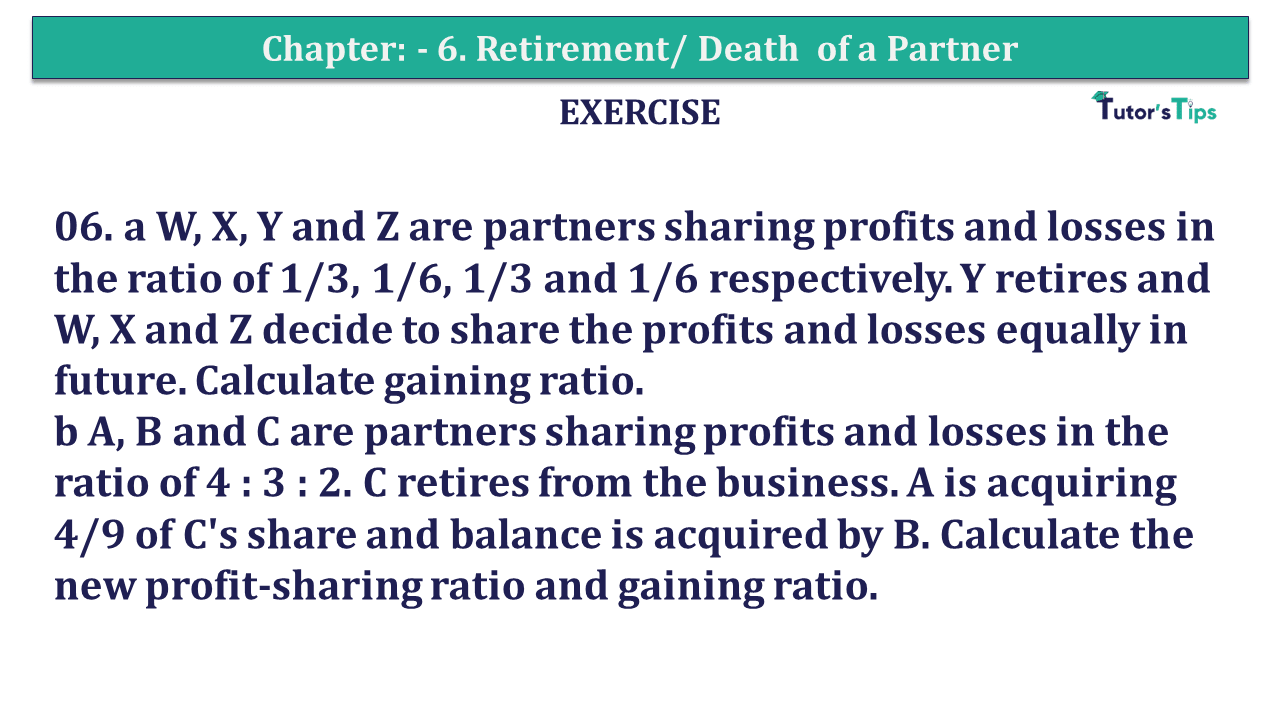# Question 06 Chapter 6 of +2-A – T.S. Grewal 12 Class Part – A Vol. 1Question No.06 Chapter No.6 - T.S. Grewal +2 Book 2019-Solution

Question 06 Chapter 6 of +2-A

06. a W, X, Y and Z are partners sharing profits and losses in the ratio of 1/3, 1/6, 1/3 and 1/6 respectively. Y retires and W, X and Z decide to share the profits and losses equally in future. Calculate gaining ratio.
b A, B and C are partners sharing profits and losses in the ratio of 4 : 3 : 2. C retires from the business. A is acquiring 4/9 of C’s share and balance is acquired by B. Calculate the new profit-sharing ratio and gaining ratio.

## The solution of Question 06 Chapter 6 of +2-A: –

a

 Old Ratio A, B and C = 1 : 3 : 1 : 1 2 10 5 6

or 2 : 1 : 2 : 1
Gaining Ratio = New Ratio − Old Ratio

 W’s Gain = 1 – 2 3 6
 = 2 – 2 6
 = 0 6

 X’s Gain = 1 – 1 3 6
 = 2 – 1 6

 = 1 6

 Z’s Gain = 1 – 1 3 6
 = 2 – 1 6
 = 1 6

∴Gaining Ratio = 0 : 1 : 1

b

Old Ratio A, B and C = 4 : 3 : 2

 C’s Profit Share = 2 9

A acquires 4/9 of C’s Share and remaining share is acquired by B

 Share acquired by A = 2 X 4 9 9
 = 8 81

Share acquired by B = C’s Share – Share acquired by A

 = 2 – 8 9 81
 = 18 – 8 81
 = 10 81

New Profit Share = Old Profit Share + Share acquired from C

 A’s new share = 4 + 8 9 81
 = 39 + 8 81
 = 44 81

 B’s new share = 3 + 10 9 81
 = 27 + 10 81
 = 37 81

∴ New Profit Ratio A and B = 44 : 37

Gaining Ratio = New Ratio − Old Ratio

 A’s new share = 44 – 4 81 9
 = 44 – 36 81
 = 8 81

 B’s new share = 37 – 3 81 9
 = 37 – 27 81
 = 10 81

∴Gaining Ratio = 8 : 10 or 4 : 5

T.S. Grewal’s Double Entry Book Keeping +2 (Vol. I: Accounting for Not-for-Profit Organizations and Partnership Firms)

• Chapter No. 1 – Financial Statement of Not-For-Profit Organisations
• Chapter No. 2 – Accounting for Partnership Firms – Fundamentals
• Chapter No. 3 – Goodwill: Nature and Valuation
• Chapter No. 4 – Change in Profit-Sharing Ratio Among the Existing Partners
• Chapter No. 5 – Admission of a Partner
• Chapter No. 6 – Retirement/Death of a Partner
• Chapter No. 7 – Dissolution of a Partnership Firm

### T.S. Grewal’s Double Entry Book Keeping (Vol. II: Accounting for Companies)

• Chapter No. 1 – Financial Statements of a Company
• Chapter No. 2 – Financial Statement Analysis
• Chapter No. 3 – Tools of Financial Statement Analysis – Comparative Statements and Common- Size Statements
• Chapter No. 4 – Accounting Ratios
• Chapter No. 5 – Cash Flow Statement